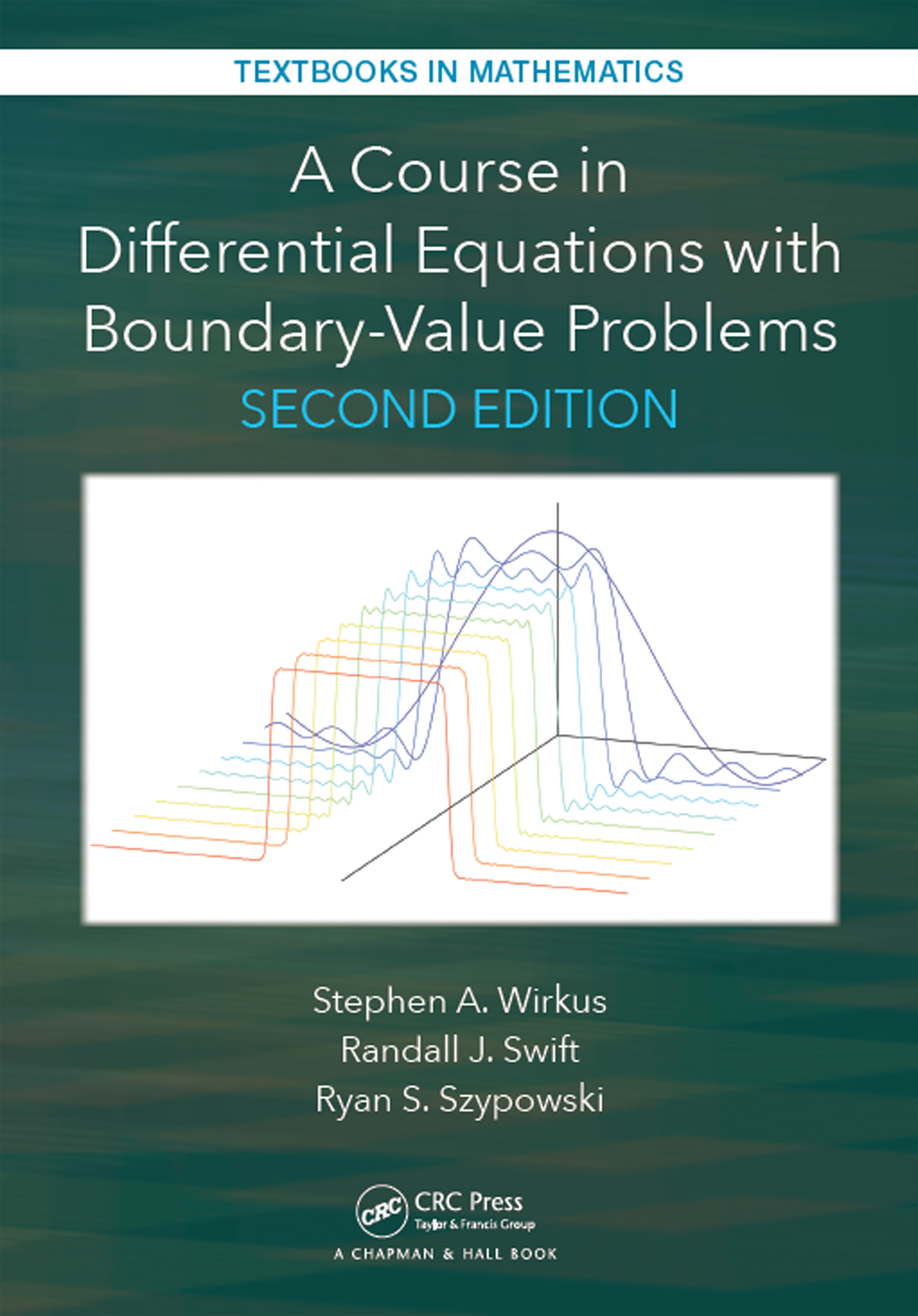# A Course in Differential Equations with Boundary Value Problems

## 2nd Edition

Chapman and Hall/CRC

768 pages | 50 B/W Illus.

##### Purchasing Options:\$ = USD
Hardback: 9781498736053
pub: 2016-12-29
SAVE ~\$26.00
\$130.00
\$104.00
x
eBook (VitalSource) : 9781315369617
pub: 2017-01-24
from \$65.00

FREE Standard Shipping!

### Description

A Course in Differential Equations with Boundary Value Problems, 2nd Edition adds additional content to the author’s successful A Course on Ordinary Differential Equations, 2nd Edition. This text addresses the need when the course is expanded.

The focus of the text is on applications and methods of solution, both analytical and numerical, with emphasis on methods used in the typical engineering, physics, or mathematics student’s field of study. The text provides sufficient problems so that even the pure math major will be sufficiently challenged.

The authors offer a very flexible text to meet a variety of approaches, including a traditional course on the topic. The text can be used in courses when partial differential equations replaces Laplace transforms. There is sufficient linear algebra in the text so that it can be used for a course that combines differential equations and linear algebra.

Most significantly, computer labs are given in MATLAB®,　Mathematica®, and Maple™. The book may be used for a course to introduce and equip the student with a knowledge of the given software. Sample course outlines are included.

Features

• MATLAB®,　Mathematica®, and Maple™ are incorporated at the end of each chapter.

All three software packages have parallel code and exercises;

• There are numerous problems of varying difficulty for both the applied and pure math major, as well as problems for engineering, physical science and other students.
• An appendix that gives the reader a "crash course" in the three software packages.
• Chapter reviews at the end of each chapter to help the students review
• Projects at the end of each chapter that go into detail about certain topics and introduce new topics that the students are now ready to see
• Answers to most of the odd problems in the back of the book

Traditional First-Order Differential Equations

Introduction to First-Order Equations

Separable Differential Equations

Linear Equations

Some Physical Models Arising as Separable Equations

Exact Equations

Special Integrating Factors and Substitution Methods

Bernoulli Equation

Homogeneous Equations of the Form g(y=x)

Geometrical and Numerical Methods for First-Order Equations

Direction Fields|the Geometry of Differential Equations

Existence and Uniqueness for First-Order Equations

First-Order Autonomous Equations|Geometrical Insight

Graphing Factored Polynomials

Bifurcations of Equilibria

Modeling in Population Biology

Nondimensionalization

Numerical Approximation: Euler and Runge-Kutta Methods

An Introduction to Autonomous Second-Order Equations

Elements of Higher-Order Linear Equations

Introduction to Higher-Order Equations

Operator Notation

Linear Independence and the Wronskian

Reduction of Order|the Case n = 2

Numerical Considerations for nth-Order Equations

Essential Topics from Complex Variables

Homogeneous Equations with Constant Coe□cients

Mechanical and Electrical Vibrations

Techniques of Nonhomogeneous Higher-Order Linear Equations

Nonhomogeneous Equations

Method of Undetermined Coe□cients via Superposition

Method of Undetermined Coe□cients via Annihilation

Exponential Response and Complex Replacement

Variation of Parameters

Cauchy-Euler (Equidimensional) Equation

Forced Vibrations

Fundamentals of Systems of Differential Equations

Useful Terminology

Gaussian Elimination

Vector Spaces and Subspaces

The Nullspace and Column Space

Eigenvalues and Eigenvectors

A General Method, Part I: Solving Systems with Real and Distinct or Complex

Eigenvalues

A General Method, Part II: Solving Systems with Repeated Real Eigenvalues

Matrix Exponentials

Solving Linear Nonhomogeneous Systems of Equations

Geometric Approaches and Applications of Systems of Differential Equations

An Introduction to the Phase Plane

Nonlinear Equations and Phase Plane Analysis

Systems of More Than Two Equations

Bifurcations

Epidemiological Models

Models in Ecology

Laplace Transforms

Introduction

Fundamentals of the Laplace Transform

The Inverse Laplace Transform

Laplace Transform Solution of Linear Differential Equations

Translated Functions, Delta Function, and Periodic Functions

The s-Domain and Poles

Solving Linear Systems Using Laplace Transforms

The Convolution

Series Methods

Power Series Representations of Functions

The Power Series Method

Ordinary and Singular Points

The Method of Frobenius

Bessel Functions

Boundary-Value Problems and Fourier Series

Two-Point Boundary-Value Problems

Orthogonal Functions and Fourier Series

Even, Odd, and Discontinuous Functions

Simple Eigenvalue-Eigenfunction Problems

Sturm-Liouville Theory

Generalized Fourier Series

Partial Differential Equations

Separable Linear Partial Differential Equations

Heat Equation

Wave Equation

Laplace Equation

Non-Homogeneous Boundary Conditions

Non-Cartesian Coordinate Systems

A An Introduction to MATLAB, Maple, and Mathematica

MATLAB

Some Helpful MATLAB Commands

Programming with a script and a function in MATLAB

Maple

Some Helpful Maple Commands

Programming in Maple

Mathematica

Some Helpful Mathematica Commands

Programming in Mathematica

B Selected Topics from Linear Algebra

A Primer on Matrix Algebra

Matrix Inverses, Cramer's Rule

Calculating the Inverse of a Matrix

Cramer's Rule

Linear Transformations

Coordinates and Change of Basis

Similarity Transformations

Computer Labs: MATLAB, Maple, Mathematica

Answers to Odd Problems

### Subject Categories

##### BISAC Subject Codes/Headings:
MAT003000
MATHEMATICS / Applied
MAT007000
MATHEMATICS / Differential Equations